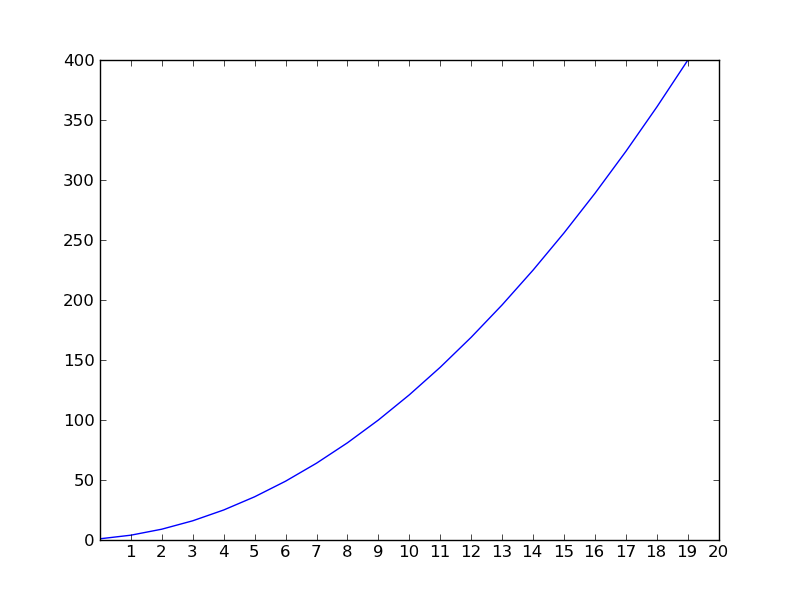# xticks() shifted by 1

Hi,

import matplotlib.pyplot as plt

d={}

for i in range(1,21):

d[i] = i**2

plt.plot(d.values())

plt.xticks(d.keys())

plt.show()

As it can be seen from attached screenshot, xticks values are shifted to right (by 1).

Seems strange, but perhaps something to do with Python 0-start.

So why plot starts at beginning of x-axis and xticks start shifted by 1?

How does regular matplotlib users handle similar code?

ThanksHi,

import matplotlib.pyplot as plt

d={}
for i in range(1,21):
d[i] = i**2

plt.plot(d.values())

This is plotting values against the zero-based index. What you want is

plt.plot(d.keys(), d.values())

···

On 09/17/2011 09:57 AM, Klonuo Umom wrote:

plt.xticks(d.keys())
plt.show()

As it can be seen from attached screenshot, xticks values are shifted to
right (by 1).

Seems strange, but perhaps something to do with Python 0-start.
So why plot starts at beginning of x-axis and xticks start shifted by 1?
How does regular matplotlib users handle similar code?

Thanks

------------------------------------------------------------------------------
BlackBerry&reg; DevCon Americas, Oct. 18-20, San Francisco, CA
http://p.sf.net/sfu/rim-devcon-copy2

_______________________________________________
Matplotlib-users mailing list
Matplotlib-users@lists.sourceforge.net
https://lists.sourceforge.net/lists/listinfo/matplotlib-users

When you called plot() with only the y values, mpl will assume x values as range(len(y)). Just call plot with both x and y values.

Ben Root

···

On Saturday, September 17, 2011, Klonuo Umom <klonuo@…287…> wrote:

Hi,
import matplotlib.pyplot as plt
d={}

for i in range(1,21):
d[i] = i**2
plt.plot(d.values())
plt.xticks(d.keys())
plt.show()

As it can be seen from attached screenshot, xticks values are shifted to right (by 1).

Seems strange, but perhaps something to do with Python 0-start.
So why plot starts at beginning of x-axis and xticks start shifted by 1?
How does regular matplotlib users handle similar code?
Thanks

While this is safe because calls to keys and values will return lists
in congruent order of no intervening dict modifications are made

http://stackoverflow.com/questions/835092/python-dictionary-are-keys-and-values-always-the-same-order

it is a bit fragile because there is no guarantee the dict keys will
be ordered, right, which is probably not what the OP wants. Maybe

x = sorted(d.keys())
y = [d[k] for k in x]
plot(x, y)

JDH

···

On Sat, Sep 17, 2011 at 3:09 PM, Eric Firing <efiring@...202...> wrote:

On 09/17/2011 09:57 AM, Klonuo Umom wrote:

Hi,

import matplotlib.pyplot as plt

d={}
for i in range(1,21):
d[i] = i**2

plt.plot(d.values())

This is plotting values against the zero-based index. What you want is

plt.plot(d.keys(), d.values())

Yes, I noticed the same and indeed I used sorted values in original problem
I forgot to add it in my simplified snippet

···

On Sat, Sep 17, 2011 at 10:34 PM, John Hunter <jdh2358@...287...> wrote:

While this is safe because calls to keys and values will return lists
in congruent order of no intervening dict modifications are made

http://stackoverflow.com/questions/835092/python-dictionary-are-keys-and-values-always-the-same-order

it is a bit fragile because there is no guarantee the dict keys will
be ordered, right, which is probably not what the OP wants. Maybe

x = sorted(d.keys())
y = [d[k] for k in x]
plot(x, y)